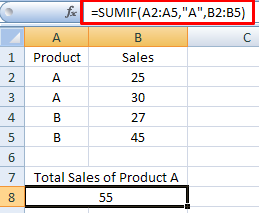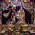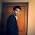# 50 Data Analyst Interview Questions

This tutorial explains common and tricky data analyst interview questions with answers. The main responsibility of data analyst is to generate insights from data and present it to stakeholders such as external or internal clients. During this process, he/she extracts data from database and then clean it up to prepare it for analysis. Later data analysis step involves exploration of data with descriptive statistics and then building predictive model for prediction. Data analyst must know basics and intermediary statistics and know how to apply it with SAS / SPSS. Excel and SQL are the two most popular tools used in data analytics so candidate must possess a good knowledge of these tools. Excel is used for a variety of purposes such as generating quick summaries and presenting it in an interactive excel dashboard. Mostly offline reporting deliverables are in either excel or powerpoint report formats.

This tutorial covers interview questions on the following topics:
1. MS Excel
2. Basic and Intermediate Statistics
3. SAS
4. SQL
5. HR / Project related questions

Excel Questions

The following is a list of some tricky or advanced excel interview questions.

1. What is the default value of last parameter of VLOOKUP?

TRUE/1 . It refers to finding the closest (approximate) match and assuming the table is sorted in ascending order. Whereas, FALSE/0 refers to exact match.

2. What is the main limitation of VLOOKUP function?

The lookup value should be at the most left side column in the table array. VLOOKUP only looks right. It cannot look right to left.

3. Does VLOOKUP look up case-sensitive values?

No, it is not case-sensitive. The text 'ram' and 'RAM' is identical for VLOOKUP.

4. 2 ways to extract unique values in excel

Use Advanced Filter option (shortcut key : ALT D F A) and 'Remove Duplicates' option under Data tab.

5. How to find duplicates in a column?

Use CONDITIONAL FORMATING to highlight duplicate values. OR use COUNTIF function as shown below. For example, values are stored in cells D4:D7.
=COUNTIF(D4:D7,D4)
Apply filter on the column wherein you applied COUNTIF function and select values greater than 1.

6. How to insert a drop down?

Go to Data tab >> Select Data Validation. Another way to insert a drop down is to enable Developer tab and Insert Combo box.

7. How to sum values based on some conditions?

Use SUMIF or SUMPRODUCT functions. The SUMIF function is explained below -
=SUMIF(range, criteria, sum_range)
=SUMIF(A2:A5,"A",B2:B5)Excel : SUMIF Function

8. How to create cross tabulation in Excel?
Use Pivot Table and select one variable in Row label and the other variable in Column label.

9. What is Excel Array Formula?

10. How to extract First Name from a full name?

Suppose you need to pull 'Neha' from 'Neha Sharma'. Use MID and FIND functions.
=MID (A2,1,FIND(" ",A2)-1)
Tutorial : Practical Uses of MID Function

11. How Index and Match Function works?

Index function returns a value from a range based on row number.
= INDEX(range, row_number)
See the image below -Excel : Index Function
In this case, we are telling EXCEL to return second value of the range A2:A4. It returns 30.

Match function returns the relative position of a value in range.
= MATCH(lookup_value, range, match_type)
match_type can be exact match, largest/smallest value that is less than or greater than equal to lookup_value.Excel : Match Function
In this case, we are asking EXCEL to find the relative position of 30 in the range A2:A4. It returns 2.

12. How Index and Match Function works together?
=INDEX(range, MATCH(lookup_value, lookup_range, match_type))
Suppose information of Product and Sales are stored in columns A and B. You need to look for product against sales value so you need to tell EXCEL to look from right to left as sales value is placed in the right hand side of the range/table.
=INDEX(A2:A5,MATCH(45,B2:B5,0))Nested INDEX MATCH Excel Functions

Basic and Intermediate Statistics

The following questions touch upon some basics and intermediate statistics topics. These topics are generally taught in undergraduate / graduate courses.

1. What is p-value?

It is the lowest level of significance at which you can reject the null hypothesis. If p-value < 0.05, you reject the null hypothesis at 5% level of significance.

2. Difference between MEAN. MEDIAN, MODE

Mean is calculated by summing all the values divided by number of observations. Median is the middle value. And Mode is the most occurring value.

3. In which data types MEAN, MEDIAN and MODE are more suitable?

MEAN is suitable for continuous data with no outliers. It is affected by extreme values (Outliers).
MEDIAN is suitable for continuous data with outliers or ordinal data. Mode is suitable for categorical data (including both nominal and ordinal data).

4. Different Types of Sampling Techniques?

There are following four main types of sampling techniques.
1. Simple random sampling
2. Stratified sampling
3. Cluster sampling
4. Systematic sampling

5. Difference between Cluster and Stratified Sampling?

The main difference between cluster and stratified sampling is that in stratified sampling all the strata need to be sampled. In cluster sampling one proceeds by first selecting a number of clusters at random and then sampling each cluster or conduct a census of each cluster. But usually not all clusters would be included.

6. When should we use T-test than Z-test?

Theoretically, we should use T-test when sample size (N) is less than 30. Practically, we always use t-test. It is because t-test and z test are equivalent as N tends to infinity.

7. What is the difference between R-square and Adjusted R-square?
Check out this link - R-square vs. Adjusted R-square

8. How to detect outliers?

Box Plot Method - If a value is higher than the 1.5*IQR above the upper quartile (Q3), the value will be considered as outlier. Similarly, if a value is lower than the 1.5*IQR below the lower quartile (Q1), the value will be considered as outlier.

Standard Deviation Method - If a value is higher than the mean plus or minus three Standard Deviation is considered as outlier.

9. Define Homoscedasticity?

In a linear regression model, there should be homogeneity of variance of the residuals. In other words, the variance of residuals are approximately equal for all predicted dependent variable values.

10. Difference between Standardized and Unstandardized Coefficients?

To calculate Standardized Coefficients, first we need to standardize both dependent and independent variables and use the standardized variables in the regression model to get standardized estimates. By 'standardize', it implies subtracting mean from each observation and divide that by the standard deviation. The standardized coefficient is interpreted in terms of standard deviation. Whereas, unstandardized coefficient is measured in actual units.

11. Difference between Factor Analysis and Principal Component Analysis?

Both the analysis are very much similar but they are different in terms of calculation and their practical usage :
1. In Principal Components Analysis, the components are calculated as linear combinations of the raw input variables. In Factor Analysis, the raw input variables are defined as linear combinations of the factors.
2. The main idea of using PCA is to explain as much of the total variance in the variables as possible. Whereas, the factor analysis explains the covariances or correlations between the variables.
3. PCA is used when we need to reduce the number of variables (dimensionality reduction) whereas FA is used when we need to group variables into some factors.

12. Difference between Linear and Logistic Regression?

There are more than 10 differences between these two algorithms. Check out the link below -

13. How to statistically compare means between groups?

Use Independent T-test when a continuous variable and a categorical variable having two independent categories.

Use Paired T-test when a continuous variable and a categorical variable having two dependent or paired categories.

Use one way ANOVA when a continuous variable and a categorical variable having more than two independent categories.

Use GLM Repeated Measures when a continuous variable and a categorical variable more than two dependent categories.

14. Explain eigenvalues and eigenvectors intuitively

Eigenvalues are variances explained by principal components. By 'variances', i mean the diagonal values of the covariance matrix below -
x y z
1.34 -0.16 0.19
-0.16 0.62 -0.13
0.19 -0.13 1.49

The sum of the diagonal values is 3.45.

Why eigenvalue greater than 1 is considered to retain components? It is because the average eigenvalue will be 1, so > 1 implies higher than average.

Eigenvectors
are the coefficients of orthogonal (uncorrelated) transformation of variables into principal components.

SAS

The following questions would help you to prepare for SAS interview round.

1. Difference between WHERE and IF statement?
• WHERE statement can be used in procedures to subset data while IF statement cannot be used in procedures.
• WHERE can be used as a data set option while IF cannot be used as a data set option.
• WHERE statement is more efficient than IF statement. It tells SAS not to read all observations from the data set
• WHERE statement can not be used when reading data using INPUT statement whereas IF statement can be used.
• When it is required to use newly created variables, use IF statement as it doesn't require variables to exist in the READIN data set.

2. How PROC MEANS works?
PROC MEANS DATA = dataset_name;
VAR analysis_variable;
CLASS grouping_variable;
RUN;
Detailed Explanation : PROC MEANS

3. Difference between INFORMAT and FORMAT?

Informat is used to read data whereas Format is used to write or display data.

4. Difference between NODUPKEY and NODUP in PROC SORT?

The NODUPKEY option removes duplicate observations where value of a variable listed in BY statement is repeated while NODUP option removes duplicate observations where values in all the variables are repeated.

5. How many maximum characters SAS library name can take?

A valid library name must start with an alphabet and cannot have more than 8 characters.

6. Which is more faster - Proc SQL or SAS data step?

The SQL procedure performed better with the smaller datasets whereas the data step performed better with the larger datasets (more than approx. 100 MB).

7. Two main advantages of Proc SQL Joins over Data Step Merging?
1. Proc SQL JOINS do not require variables to be sorted prior to joining them whereas Data Step Merging requires.
2. Proc SQL works perfectly when key variables have different names

8. What would happen if i don't use 'BY statement in MERGE?

Without 'BY' statement, SAS will merge the 1st observation from dataset A and 1st observation from dataset B to form the 1st observation of the final dataset. It might lead to meaningless results.

9. What are the ways to create a macro variable?

There are 5 ways to create macro variables:
• %Let
• Iterative %DO statement
• Call Symput
• Proc SQl into clause
• Macro Parameters

10. How to rename columns with PROC SQL?

Use AS alias.
Proc SQL;
select name as fullname from table1;
quit;

11. How to calculate percentile values with SAS?

We can use PROC MEANS or PROC UNIVARIATE to calculate percentile values. For example, specify options P10, P90 to calculate 10th and 90th percentile score. PROC MEANS cannot calculate custom percentile values such as 97.5th or 99.5th percentile. To calculate these custom percentile values, you can use PCTLPTS= option in PROC UNIVARIATE.

Tutorial : PROC UNIVARIATE

12. How to replace missing values of all the numeric variables to 0 in a single run?

We can use _numeric_ to specify all the numeric variables and dim functions in array to count the number of numeric variables.
data temp;
set sampledata;
array Q(*) _numeric_;
do i= 1 to dim(Q);
if Q(i) = . then Q(i)= 0;
end;
run;

SQL

1. How to write conditional statements (IF ELSE) in SQL?

In SQL, it is possible with CASE WHEN statements.
select
case when sex='M' then 1 else 0 end as males
, case when sex='F' then 1 else 0 end as females
from sashelp.class;
quit;

2. What are the common SQL data types ?

Data Type Format
Numeric NUMBER(L,D), INTEGER,SMALLINT,DECIMAL(L,D)
Character CHAR(L),VARCHAR(L)
Date DATE

3. How to subset or filter data in SQL?

We can use WHERE clause to subset or filter data.
SELECT *
FROM PRODUCT
WHERE SALES > 200

4. Difference between WHERE and HAVING clauses

The HAVING clause comes into effect when GROUP BY is used. It runs after GROUP BY so it filters grouping variable. Whereas WHERE clause runs prior to GROUP BY clause so it does not filter a grouping variable.

5. Difference between Full Join and Cross Join?

A full join keeps all rows from both of the input tables even if we cannot find a matching row.
Cross Join returns cartesian product of tables. It matches every row of one table with every row of another table.

6. Difference between UNION and UNION ALL

The main use of UNION and UNION ALL is to join two tables. The main difference between them is that UNION removes duplicate records and UNION ALL keeps the duplicate records. By 'duplicate records', all the values of two or more rows are same.

7. How to create a blank table

Method I :

The following method creates a new table called temp2 with the same column names and attributes of table temp.
CREATE TABLE TEMP2 LIKE TEMP;
Method II :

In this case, we are creating a blank table by subsetting data method. As 1 is not equal to 2, it returns zero row.
create table temp3 as
select * from temp
where 1=2;

8. What will be the result of the query below?
select case when null = null then 'Yes' else 'No' end as Result;
It will return NO as the above code is not right way to compare null values. The correct way would be to use 'is' keyword to compare -
select case when null is null then 'Yes' else 'No' end as Result;

9. Suppose you have a table named TEMP. You need to recode values of column Y, Swap values 2 and 3 in column YTable

UPDATE TEMP
SET Y= CASE WHEN Y = 2 THEN 3
WHEN Y = 3 THEN 2
ELSE Y END;

10. Identify second maximum value
select max(y) from temp
where y not in (select max(y) from temp);
In this code, the logic is to remove the maximum value from the main table and then calculate the max value to figure out the second max value of the main table.

11. Identify second maximum value by a group

The code below first removes all the max values by a group from the main table. Later we calculated the second max value by a group.
select a.x, max(a.y) as maxy from temp a left join
(select x, max(y) as maxy from temp group by 1) b
on a.x = b. x and a.y = b.maxy
where b.x is null and b.maxy is null
group by 1;

12. Is the query below correct? If not, what's the issue?
SELECT custid, YEAR(ref_date) AS ref_year
FROM custmart
WHERE ref_year  >= 2015;
The calculated column cannot be used in WHERE condition so we need to modify the above code like this -
SELECT custid, YEAR(ref_date) AS ref_year
FROM custmart
WHERE YEAR(ref_date)  >= 2015;

HR / Project Related Questions

1. Explain one of your project

• Start from problem definition
• Explain Data Cleaning, Exploration and Data Preparation Steps
• What technique / algorithm is used in the project?
• Financial (Dollar) value impact of the project

2. What are your strengths and weaknesses?

3. Why are you leaving the current organization?

4. Why should we hire you?

5. Where do you see yourself five years from now?

6. What was the toughest decision you ever had to make?

End Notes

The above list of questions would assist you in preparing for interviews for roles of senior / lead data analyst. Don't just mug up answers, understand the concepts of the topics covered in these questions.Related PostsDeepanshu founded ListenData with a simple objective - Make analytics easy to understand and follow. He has over 8 years of experience in data science. During his tenure, he has worked with global clients in various domains like Banking, Insurance, Telecom and Human Resource.

6 Responses to "50 Data Analyst Interview Questions"
1.Excellent blog

2.Please add some more question related to excel and sas base

3.extremely helpful and up to the mark blog. great job

4.3rd Answer is wrong. It should be 'Yes' cos its case sensitive.

1.It is not case-sensitive. can you try it once using excel?

5.Fan Ho gaya sir me ap ka!!

Next → ← Prev
Love this Post? Spread the Word!
Share